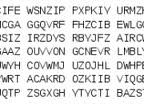## Brute Forcing One Time Pads - Defcon Mystery Challenge Cryptanalysis PreparationIn preparation for the mystery challenge at Defcon 20 this year, I put together some simple python tools.  I find it quite enjoyable making anti-1057 cryptanalysis tools, so I may be adding more.  This is what I have so far.

Update: This is now on Github

## Generate Lookups

Each of these lookups is a dictionary.  The key:value pairs in each dictionary can easily be extended and fed into the crypto functions.

``lookup1a = {i : chr(64+i) for i in range(1,27)} #1:A, 2:B, etclookupa1 = {chr(64+i):i for i in range(1,27)} #A:1, B:2, etclookup0a = {i : chr(65+i) for i in range(0,26)} #0:A, 1:B, etclookupa0 = {chr(64+i):i-1 for i in range(1,27)} #A:0, B: 1, etc` `

The next two functions with encrypt a message and decrypt a message with the OTP modular arithmetic method.  I kept them as two separate functions to provide quick and easy customization.

`` def otp_encrypt(cleartext, key, lookup_fwd, lookup_rev):  "sample usage: ciphertext = otp_encrypt('HELLO', 'XMCKL', lookupa0, lookup0a)"  ciphertext=''  for idx, val in enumerate(cleartext):    msg_key = lookup_fwd[val] + lookup_fwd[key[idx]]    modded = msg_key % 26    ciphertext+=lookup_rev[modded]  return ciphertextdef otp_decrypt(ciphertext, key, lookup_fwd, lookup_rev):  """sample usage: cleartext = otp_encrypt('EQNVZ','XMCKL', lookupa0, lookup0a)  key must be >= len(ciphertext)"""  cleartext=''  for idx, val in enumerate(ciphertext):    cipher_key = lookupa0[val] - lookupa0[key[idx]]    modded = cipher_key % 26    cleartext+=lookup0a[modded]  return cleartext``

## Brute Force an OTP

Here is the interesting part.  What if you need to brute force a OTP because the key is unknown?  You may have a key within a larger boy of text, but the index is unknown.  For instance, assume the key is somewhere within a known body of text:

``````key = """ISOLVEMYPROBLEMSANDISEETHELIGHTWEGOTTAPLUGANDTHINKWEGOTTAFEEDITRIGHTTHEREAINTNODANGERWECANGOTOFARWESTARTBELIEVINGNOWTHATWECANBEWHOWEAREGREASEISTHEWORDTHEYTHINKOURLOVEISJUSTAGROWINGPAINWHYDONTTHEYUNDERSTANDITSJUSTACRYINGSHAMETHEIRLIPSARELYINGONLYREALISREALWESTARTTOFINDRIGHTNOWWEGOTTOBEWHATWEFEELGREASEISTHEWORDGREASEISTHEWORDISTHEWORDTHATYOUHEARDITSGOTGROOVEITSGOTMEANINGGREASEISTHETIMEISTHEPLACEISTHEMOTIONGREASEISTHEWAYWEAREFEELINGWETAKETHEPRESSUREANDWETHROWAWAYCONVENTIONALITYBELONGSTOYESTERDAYTHEREISACHANCETHATWECANMAKEITSOFARWESTARTBELIEVINGNOWTHATWECANBEWOWEAREGREASEISTHEWORDGREASEISTHEWORDISTHEWORDTHATYOUHEARDITSGOTGROOVEITSGOTMEANINGGREASEISTHETIMEISTHEPLACEISTHEMOTIONGREASEISTHEWAYWEAREFEELINGTHISISTHELIFEOFILLUSIONWRAPPEDUPINTROUBLELACEDWITHCONFUSIONWHATWEDOINGHEREWETAKETHEPRESSUREANDWETHROWAWAYCONVENTIONALITYBELONGSTOYESTERDAYTHEREISACHANCETHATWECANMAKEITSOFARWESTARTBELIEVINGNOWTHATWECANBEWHOWEAREGREASEISTHEWORDGREASEISTHEWORDISTHEWORDTHATYOUHEARDITSGOTGROOVEITSGOTMEANINGGREASEISTHETIMEISTHEPLACEISTHEMOTIONGREASEISTHEWAYWEAREFEELINGGREASEISTHEWORDISTHEWORDTHATYOUHEARDITSGOTGROOVEITSGOTMEANINGGREASEISTHETIMEISTHEPLACEISTHEMOTIONGREASEISTHEWAYWEAREFEELING"""

ciphertext = "XTEZPTPRPBFGTBOJPKYEAAZPXOBFTYAPWMERMMSDBFJGQUGBAMHNWSEKTSPVIVFAXFJ"
````chaff=''for i in range(len(key)-len(ciphertext)):  chaff+= otp_decrypt(ciphertext,key[i:], lookupa0, lookup0a)+'\n'#write each decryption attempt to a line & output to a file for easy viewing/searching/greppingofp = open('out.txt','w')   ofp.write(chaff)````

It would be nifty to compare the chaff outputted here with an english dictionary.

## Caeser Cipher Brute Forcer

Since this one is simple, I am going to include it too.  If you have some ciphertext where you want to quickly see all 26 rotated varients, this function works like a charm:

``def caeser_brute(text):  'this function rot1-rot25s the input text (& converts to uppercase)'  for r in range(27):    print "\n %s - " % r,    for i in text.upper(): print chr((((ord(i)-65+r)%26)+65)),``

## In Closing

The nature of these challenges makes preparation extremely difficult.  Solutions typically require some level of customization to classical cryptography cryptanalysis methods.  The most difficult part is usually figuring out the type of cipher, identifying the plaintext and identifying the ciphertext.  The challenges usually start you in the middle and you need to determine the question.  Wish me luck!

## Other useful cryptanalysis tools

• The On-Line Encyclopedia of Integer Sequences (OEIS)
• Rumkin Cipher Tools (rumkin.com)
• Cryptool Versions 1 and 2 (cryptool.org) - offline tools, each version is different